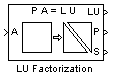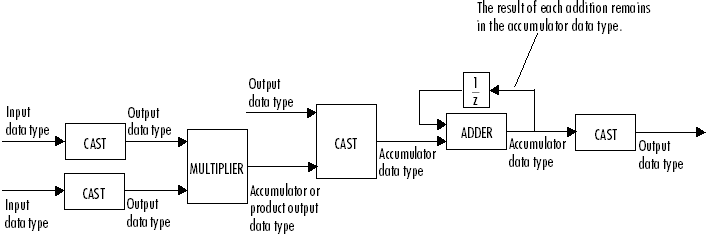Documentation

# LU Factorization

Factor square matrix into lower and upper triangular components

## Library

Math Functions / Matrices and Linear Algebra / Matrix Factorizations

`dspfactors`## Description

The LU Factorization block factors a row-permuted version of the square input matrix A as Ap = L*U, where L is a unit-lower triangular matrix, U is an upper triangular matrix, and Ap contains the rows of A permuted as indicated by the permutation index vector P. The block uses the pivot matrix Ap instead of the exact input matrix A because it improves the numerical accuracy of the factorization. You can determine the singularity of the input matrix A by enabling the optional output port S. When A is singular, the block outputs a `1` at port S; when A is nonsingular, it outputs a `0`.

To improve efficiency, the output of the LU Factorization block at port LU is a composite matrix containing both the lower triangle elements of L and the upper triangle elements of U. Thus, the output is in a different format than the output of the MATLAB® `lu` function, which returns L and U as separate matrices. To convert the output from the block's `LU` port to separate L and U matrices, use the following code:

```L = tril(LU,-1)+eye(size(LU)); U = triu(LU); ```

If you compare the results produced by these equations to the actual output of the MATLAB `lu` function, you may see slightly different values. These differences are due to rounding error, and are expected.

See the `lu` function reference page in the MATLAB documentation for more information about LU factorizations.

### Fixed-Point Data Types

The following diagram shows the data types used within the LU Factorization block for fixed-point signals.You can set the product output, accumulator, and output data types in the block dialog as discussed below.

The output of the multiplier is in the product output data type when the input is real. When the input is complex, the result of the multiplication is in the accumulator data type. For details on the complex multiplication performed, see Multiplication Data Types.

## Examples

The row-pivoted matrix Ap and permutation index vector P computed by the block are shown below for 3-by-3 input matrix A.

The `LU` output is a composite matrix whose lower subtriangle forms L and whose upper triangle forms U.See Matrix Factorizations in the DSP System Toolbox™ User's Guide for another example using the LU Factorization block.

## Parameters

Main Tab

Show singularity status

Select to output the singularity of the input at port S, which outputs Boolean data type values of 1 or 0. An output of 1 indicates that the current input is singular, and an output of 0 indicates the current input is nonsingular.

Data Types Tab

Rounding mode

Specify the rounding mode for fixed-point operations as one of the following:

• `Floor`

• `Ceiling`

• `Convergent`

• `Nearest`

• `Round`

• `Simplest`

• `Zero`

For more details, see rounding mode.

Saturate on integer overflow

When you select this parameter, the block saturates the result of its fixed-point operation. When you clear this parameter, the block wraps the result of its fixed-point operation. For details on `saturate` and `wrap`, see overflow mode for fixed-point operations.

Product output

Specify the product output data type. See Fixed-Point Data Types and Multiplication Data Types for illustrations depicting the use of the product output data type in this block. You can set it to:

• A rule that inherits a data type, for example, `Inherit: Inherit via internal rule`. For more information on this rule, see Inherit via Internal Rule.

• A rule that inherits a data type, for example, `Inherit: Same as input`.

• An expression that evaluates to a valid data type, for example, `fixdt(1,16,0)`

Click the buttonto display the Data Type Assistant, which helps you set the Product output parameter.

Accumulator

Specify the accumulator data type. See Fixed-Point Data Types for illustrations depicting the use of the accumulator data type in this block. You can set this parameter to:

• A rule that inherits a data type, for example, `Inherit: Inherit via internal rule`. For more information on this rule, see Inherit via Internal Rule.

• A rule that inherits a data type, for example, `Inherit: Same as input`.

• A rule that inherits a data type, for example, ```Inherit: Same as product output```.

• An expression that evaluates to a valid data type, for example, `fixdt(1,16,0)`

Click the buttonto display the Data Type Assistant, which helps you set the Accumulator parameter.

Output

Specify the output data type. See Fixed-Point Data Types for illustrations depicting the use of the output data type in this block. You can set it to:

• A rule that inherits a data type, for example, `Inherit: Same as input`

• An expression that evaluates to a valid data type, for example, `fixdt(1,16,0)`

Click the buttonto display the Data Type Assistant, which helps you set the Output parameter.

Lock data type settings against changes by the fixed-point tools

Select this parameter to prevent the fixed-point tools from overriding the data types you specify on the block mask.

## References

Golub, G. H., and C. F. Van Loan. Matrix Computations. 3rd ed. Baltimore, MD: Johns Hopkins University Press, 1996.

## Supported Data Types

PortSupported Data Types

A

• Double-precision floating point

• Single-precision floating point

• Fixed point (signed only)

• 8-, 16-, and 32-bit signed integers

LU

• Double-precision floating point

• Single-precision floating point

• Fixed point (signed only)

• 8-, 16-, and 32-bit signed integers

P

• Double-precision floating point

• Single-precision floating point

• 32-bit unsigned integers

S

• Boolean

Watch now